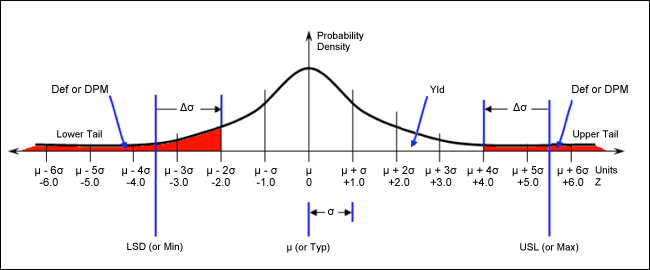# Statistical Process Control Calculator Tutorial

### 要約

The Statistical Process Control Calculator (SPCC) aids in the prediction and analysis of process yield. The calculator can be used with an HP® 50g calculator or a free PC emulator.

### Introduction

Steve Edwards, an experienced analog design engineer, has written several calculators to automate repetitive tasks. These tools are being shared to help other analog design engineers who select, specify, and characterize analog circuits. We will summarize the functionality of one such tool, the Statistical Process Control Calculator.

### Statistical Process Control Calculator

The Statistical Process Control Calculator (SPCC) is a program for the HP 50g calculator that aids in the prediction and analysis of process yield. Each parameter can be entered or found. SPCC can also be run on a PC using the free program HPUserEdit 5.4, found at www.hpcalc.org, or at Steve's Analog Design Calculators page.

Statistical process control (SPC) is a structured methodology for continuous process improvement. The ultimate goal of SPC is to achieve Six-Sigma-quality standards over the short term, as these standards are assumed to produce long-term defect levels below 3.4 defects per million (DPM). This is because it is assumed there is a 1.5σ long-term process shift, Δσ.

SPCC can calculate long-term production yield and all related parameters. It is based on the normal (sometimes called "Gaussian") distribution curve that results from the presence of random variables found in all processes and measurements.The following eight parameters can be entered or found:

1. Defects per million, DPM, in PPM

1. Or long-term yield, Yld, in %
2. Or long-term defects, Def, in %
2. Long-term process shift, Δσ, in Z
3. Short-term standard deviation, s, in user units, U
4. Short-term mean, µ, in user units, U
5. Lower specification limit, LSL, in user units, U
6. Lower specification limit, LSL, in Z
7. Upper specification limit, USL, in user units, U
8. Upper specification limit, USL, in Z

SPCC can find any parameter as a function of the others, making it useful for both predicting yield and for finding the conditions necessary to produce a desired yield. It also allows one to plot the probability density of a population based on these parameter values.

These parameters appear in the SPCC as shown below:By default, SPCC uses standard SPC terminology of µ, LSL, and USL. Alternatively, Typ, Min, and Max can be used, which are commonly found in the electrical specification of integrated circuits and other electronic components.

The user's guide (available as part of the SPCC Program Package) provides instructions for the calculator's use, the theory and equations behind the prediction and analysis of process yield, and most importantly, a practical example of its use in circuit design and analysis. The practical example will use SPCC to predict the short- and long-term production yields of an amplified pressure sensor due to variations in its output current (IOUT).